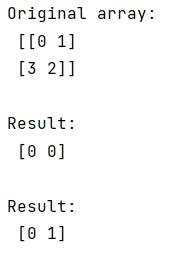# Python - How to return all the minimum indices in NumPy?

Learn, how to return all the minimum indices in Python NumPy?
Submitted by Pranit Sharma, on March 25, 2023

## Returning all the minimum indices in NumPy

Suppose that we are given a numpy array that contains some numerical values and we need to get the indices of the minimum value of the array. The minimum values are present more than once in the array at different positions.

To return all the minimum indices in NumPy, we use numpy.argmin() which returns exactly what we want in this problem. It returns the indices of the minimum values along an axis. It accepts an input array (a), axis (along which we need to apply this function), and out (location to store the result) as parameters.

Let us understand with the help of an example,

## Python code to return all the minimum indices in NumPy

```# Import numpy
import numpy as np

# Creating a numpy array
arr = np.array([[0, 1],[3, 2]])

# Display original array
print("Original array:\n",arr,"\n")

# Returning minimum indices along axis 0
res = np.argmin(arr,axis=0)

# Display result
print("Result:\n",res,"\n")

# Returning minimum indices along axis 1
res = np.argmin(arr,axis=1)

# Display result
print("Result:\n",res,"\n")
```

### Output